﻿ 基于机器视觉技术的小米品质检测方法研究

基于机器视觉技术的小米品质检测方法研究Research on Detection Method of Millet Quality Based on Machine Vision Technology

Abstract: In order to study the rapid detection and identification of millet quality, taking the latest millet and aging millet of the “Yugu 18” as the research object, the RGB image of each sample is captured by an industrial camera, and the same size region of interest (ROI) image block is cropped by image processing. Since the latest millet and aging millet differ greatly in color, in order to extract the color features better, the RGB image is converted to the HSV color space and the central moment corresponding to the hue (H) and saturation (S) of the ROI region under the HSV color space is extracted. In terms of the eigenvalue distribution map, feature vectors with distinct features are selected as input of classifier, and a detection model based on support vector machine (SVM) is established. Training and testing the model by leave-one-out cross-validation method, the results show that when the center moment feature of saturation (S) is selected as the input model of eigenvector, the recognition rate can reach 95% and it takes less time, which can be applied to millet quality detection.

1. 引言

2. 材料与方法

2.1. 材料与设备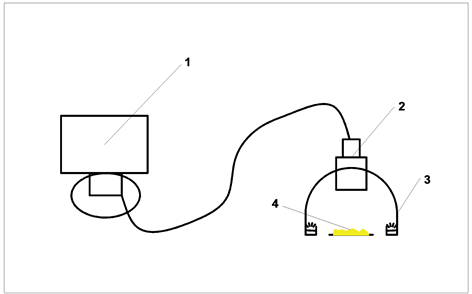1. 电脑 2. CCD工业相机 3. 漫反射圆顶光源 4. 物料样品

Figure 1. Image acquisition system

2.2. 图像采集与预处理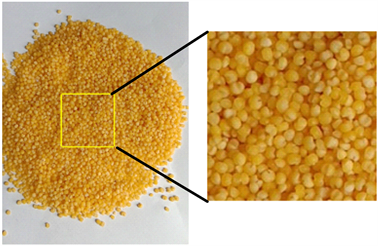(a) 原始采集图像 (b) 提取标准块图像

Figure 2. Acquisition of sample images and acquisition of standard image blocks

2.3. 颜色模型的选择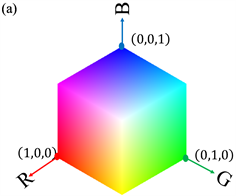(a) RGB颜色空间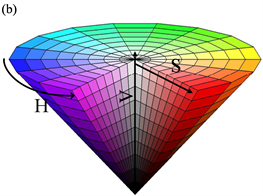(b) HSV颜色空间

Figure 3. Color space model

2.4. 图像特征提取

2.4.1. 颜色模型转换

$\begin{array}{l}\text{H}=\left\{\begin{array}{c}\frac{\text{G}-\text{B}}{\text{MAX}-\text{MIN}}×60˚,\\ \left(2+\frac{\text{B}-\text{R}}{\text{MAX}-\text{MIN}}\right)×60˚,\\ \left(4+\frac{\text{R}-\text{G}}{\text{MAX}-\text{MIN}}\right)×60˚,\end{array}\\ \text{ }\text{ }\text{ }\text{ }\text{ }\text{ }\text{ }\text{ }\text{ }\text{ }\text{ }\text{ }\text{ }\text{ }\text{ }\text{ }\text{ }\text{ }\text{ }\text{ }\text{ }\text{ }\text{ }\text{ }\text{ }\text{ }\text{ }\text{ }\text{ }\text{ }\text{ }\left(\text{R}=\text{MAX}\right)\\ \text{ }\text{ }\text{ }\text{ }\text{ }\text{ }\text{ }\text{ }\text{ }\text{ }\text{ }\text{ }\text{ }\text{ }\text{ }\text{ }\text{ }\text{ }\text{ }\text{ }\text{ }\text{ }\text{ }\text{ }\text{ }\text{ }\text{ }\text{ }\text{ }\text{ }\left(\text{G}=\text{MAX}\right)\\ \text{ }\text{ }\text{ }\text{ }\text{ }\text{ }\text{ }\text{ }\text{ }\text{ }\text{ }\text{ }\text{ }\text{ }\text{ }\text{ }\text{ }\text{ }\text{ }\text{ }\text{ }\text{ }\text{ }\text{ }\text{ }\text{ }\text{ }\text{ }\text{ }\text{ }\left(\text{B}=\text{MAX}\right)\end{array}$ (1)

$\text{S}=\frac{\text{MAX}-\text{MIN}}{\text{MAX}}$ (2)

$\text{V}=\text{MAX}$ (3)

2.4.2. 色调和饱和度直方图特征

$p\left({z}_{i}\right)=\frac{{z}_{i}}{M}$ (4)

2.4.3. 颜色直方图特征提取

${\mu }_{1}=\underset{i=0}{\overset{N-1}{\sum }}{z}_{i}p\left({z}_{i}\right)$ (5)

${\mu }_{2}=\underset{i=0}{\overset{N-1}{\sum }}{\left({z}_{i}-{\mu }_{1}\right)}^{2}p\text{\hspace{0.17em}}\left({z}_{i}\right)$ (6)

${\mu }_{3}=\underset{i=0}{\overset{N-1}{\sum }}{\left({z}_{i}-{\mu }_{1}\right)}^{3}p\text{\hspace{0.17em}}\left({z}_{i}\right)$ (7)

2.4.4. 分类模型的建立

3. 结果与分析

3.1. 数据集的划分

3.2. 实验结果与分析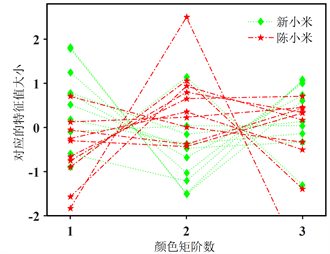(a) 色调(H)颜色矩(b) 饱和度(S)颜色矩

Figure 4. New millet and old millet color eigen value distributionTable 1. The result of classification and recognition of millet image features

4. 讨论与结论

NOTES

*通讯作者。

 陈相艳. 我国小米加工产业现状及发展趋势[J]. 农产品加工(学刊), 2011(7): 131-133.

 钱丽丽, 宋雪健, 类彦波, 王月, 左锋, 张丽媛. 近红外漫反射光谱技术对小米产地的快速检测[J]. 食品工业, 2018, 39(6): 257-261.

 张敏, 刘辉. 基于主成分分析法的小米食用品质评价模型的建立[J]. 东北农业大学学报, 2011, 42(8): 7-12.

 阎聪, 朱荣光, 黄昆鹏. 光学在线检测技术在食品品质检测中的应用[J]. 食品工业, 2018, 39(4): 292-296.

 张琛, 房胜, 王风云, 等. 基于机器学习的免套袋苹果缺陷分级[J]. 河南农业科学, 2019, 48(4): 154-160.

 Yang, G.L., Luo, L., Feng, Y.Q., et al. (2014) Research of Navel Orange Defect and Color Detection Based on Machine Vision. Applied Mechanics and Materials, 513-517, 3442-3445.
https://doi.org/10.4028/www.scientific.net/AMM.513-517.3442

 张凯, 李振华, 郁豹, 胡安翔. 基于机器视觉的花生米品质分选方法[J]. 食品科技, 2019(5): 297-302.

 李庆中, 张漫, 汪懋华. 基于遗传神经网络的苹果颜色实时分级方法[J]. 中国图象图形学报, 2000, 5(9): 71-76.

 Noordam, J.C., Otten, G.W., Timmermans, T.J.M., et al. (2000) High-Speed Potato Grading and Quality Inspection Based on a Color Vision System. Machine Vi-sion Applications in Industrial Inspection VIII, Volume 3966, 206-217.
https://doi.org/10.1117/12.380075

 张书慧, 陈晓光, 张晓梅, 等. 苹果、桃等农副产品品质检测与分级图像处理系统的研究[J]. 农业工程学报, 1999, 15(1): 201-204.

 孙海峰, 王丽霞, 王玉文, 李文德. 小米陈化过程中脂肪氧合酶与黄色素变化特征的研究[J]. 中国粮油学报, 2008, 23(6): 93-97.

 杨延兵, 管延安, 秦岭, 石慧, 王海莲, 张华文. 不同地区谷子小米黄色素含量与外观品质研究[J]. 中国粮油学报, 2012, 27(1): 14-19.

 候蕊. 谷子脂氧合酶(LOX)与小米储藏过程中褪色关系的研究[D]: [硕士学位论文]. 晋中: 山西农业大学, 2016.

 罗雪宁, 彭云发, 代希君, 等. 基于MATLAB的红枣图像处理研究[J]. 农机化研究, 2015, 37(3): 183-186.

 徐贵力, 毛罕平, 李萍萍. 彩色图像颜色和纹理特征提取的应用算法[J]. 计算机工程, 2002, 28(6): 25-27.

 王彦林. 基于HSV颜色特征图像检索算法在Matlab中的实现[J]. 电脑编程技巧与维护, 2013(16): 86-87.

 Li, B. (2009) Illumination-Independent Descriptors Using Color Moment Invariants. Optical En-gineering, 48, Article ID: 027005.
https://doi.org/10.1117/1.3083400

 张铮. 精通Matlab数字图像处理与识别[M]. 北京: 人民邮电出版社, 2013.

 姚峰林. 数字图像处理及在工程中的应用[M]. 北京: 北京理工大学出版社, 2014.

 Gonzalez, R.C., et al. 数字图像处理的MATLAB实现[M]. 北京: 清华大学出版社, 2013.

 Gonzalez, R., Woods, R. and Eddins, S. (2013) Digital Image Processing Using MATLAB. Electronic In-dustry Press, Beijing.

 梁韶聪, 周明, 李安安. 基于步态能量图的KPCA和SVM的步态识别方法[J]. 计算机应用研究, 2010, 27(7): 2798-2800.

 王蒙军. 唇读发声器中视觉信息的检测与处理[D]: [博士学位论文]. 天津: 天津大学, 2007.

 徐爽, 何建国, 贺晓光, 等. 基于高光谱技术的长枣内外品质同时检测[J]. 光电子•激光, 2013(10): 1972-1976.

Top